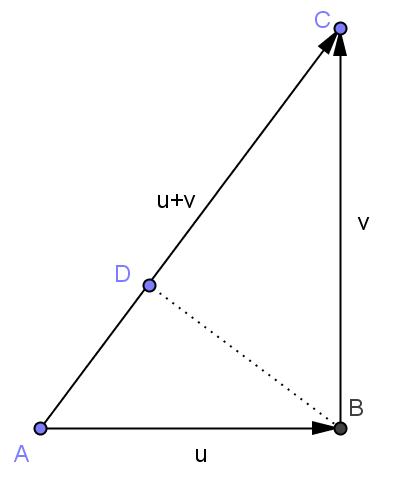# 平行四边形法则与勾股定理–内积与范数

1) $$\forall v\in V,|v|\ge 0$$，并且 $$|v|=0$$ 当且仅当 $$v=0$$。
2) $$|av|=|a| |v|$$
3) $$|u+v|\le |u|+|v|$$

# 用向量的内积证明勾股定理——体会代数的威力

||u+v||²=<u+v,u+v>=<u,u+v>+<v,u+v>=<u,u>+<u,v>+<v,u>+<v,v>。4月27日有关余弦定理的补充：

||u-v||²=<u-v,u-v>=<u,u>+<v,v>-<u,v>-<v,u>=||u||²+||v||²-2<u,v>
||u+v||²=<u+v,u+v>=<u,u>+<v,v>+<u,v>+<v,u>=||u||²+||v||²+2<u,v>

<u,v>=<v,u>=0，和勾股定理||u-v||²=||u+v||²=||u||²+||v||²